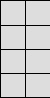Name:    Chapter 6 Test

Multiple Choice
Identify the choice that best completes the statement or answers the question.

Find the surface area of the figure.

1.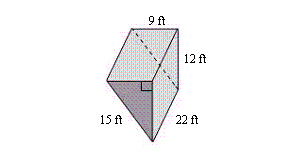a. 900 ftc. 1008 ftb. 1692 ftd. 896 ft2.

What is the least amount of cardboard needed to make a box that measures 8 inches by 12 inches by 12 inches?
 a. 672 in.c. 1152 in.b. 336 in.d. 528 in.Find the surface area of the figure.

3.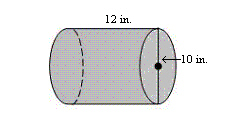a. 1004.8 in.c. 816.4 in.b. 533.8 in.d. 910.6 in.Use the net to find the surface area of the regular pyramid.

4.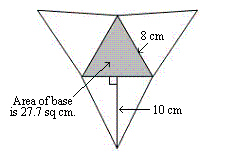a. 240 cmc. 147.7 cmb. 267.7 cmd. 120 cm5.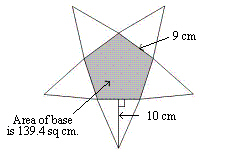a. 225 cmc. 589.4 cmb. 450 cmd. 364.4 cmFind the surface area of the figure.

6.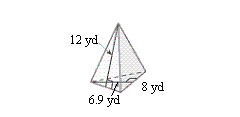a. 171.6 ydc. 315.6 ydb. 288 ydd. 144 yd7.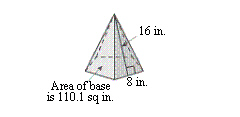a. 750.1 in.c. 640 in.b. 320 in.d. 430.1 in.Find the surface area of the figure.

8.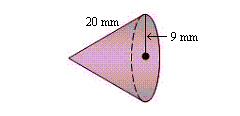a. 565.2 mmc. 813.3 mmb. 1384.7 mmd. 819.5 mm9.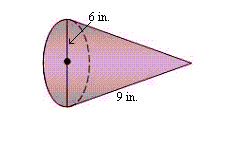a. 113 in.c. 169.6 in.b. 104.5 in.d. 197.8 in.Find the slant heightof the cone.

10.

S = 192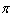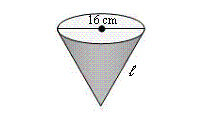a. 16 cm c. 19 cm b. 17 cm d. 2.7 cm

11.

S = 48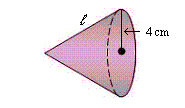a. 5 cm c. 2.4 cm b. 6 cm d. 8 cm

Find the surface area of the figure.

12.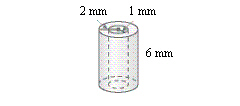a. 144.44 mmc. 119.32 mmb. 94.2 mmd. 131.88 mm13.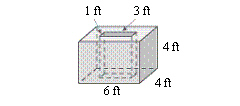a. 154 ftc. 157 ftb. 160 ftd. 138 ftNumeric Response

1.

The surface area of a square pyramid is 95 square feet. The base length is 5 feet. What is the slant height?

2.

A company packages frozen food cones. How many square inches of paper are needed to completely enclose one food cone?  Round to the nearest centimeter.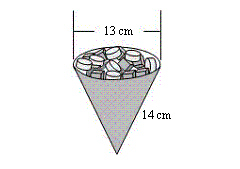front: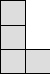top: